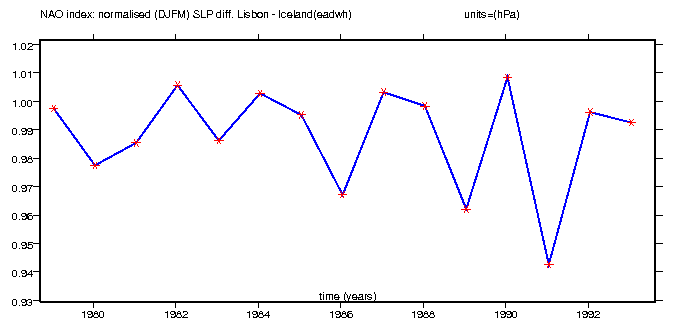## North Atlantic Oscillation (NAO)

The North Atlantic Oscillation Index presented here is a function of the difference between the surface level pressure (SLP) in Akuyreyr, Iceland and the SLP in Lisbon, Portugal. Following Hurrell (1995).

To calculate the NAO index the winter SLP anomalies are normalised by dividing the measured seasonal pressure values by the long-term standard deviation of the seasonal sea level pressure. (This prevents the NAO index from being skewed towards the greater variability of the Icelandic low).

The SLP data presented here are NOT normalised if the length of the simulation is less than 10 years. Instead the time series plot simply shows the difference in pressure between Lisbon and Iceland.

Python algorithms for Iceland and Lisbon surface level pressure:
slp_iceland=av(var_slp(lat=(64.6, 66.6), lon=(340.9,342.9), squeeze=1),axis="xy")
slp_lisbon=av(var_slp(lat=(37.8, 39.8), lon=(349.9, 351.9), squeeze=1), axis="xy")

See Jim Hurrell's web page for further details on NAO indices.NAO index as a function of Lisbon minus Iceland SLP. Lisbon (38.8N,350.9E) Iceland (65.6N, 341.9E) gif ps